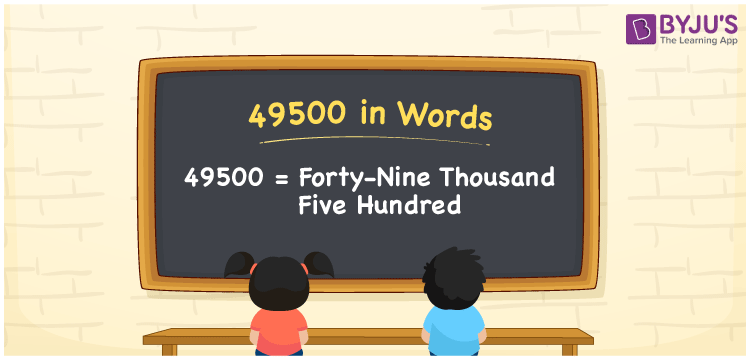# 49500 in Words

49500 in words is written as Forty-nine thousand five hundred. In both the International System of Numerals and the Indian System of Numerals, 49500 is written as Forty-nine thousand five hundred. The number 49500 is a Cardinal Number as it could represent some quantity. For example, “that laptop costs 49500 rupees”.

 49500 in words Forty-nine thousand five hundred Forty-nine thousand five hundred in Number 49500

## 49500 in English Words

49500 in English words is read as “Forty-nine thousand five hundred.”## How to Write 49500 in Words?

To write 49500 in words, we shall use the place value chart. In the place value chart, put 4 in the ten thousands, 9 in the thousands, 5 in the hundreds and put 0 in the rest. Let us make a place value chart to write the number 49500 in words.

 Ten Thousands Thousands Hundreds Tens Ones 4 9 5 0 0

Thus, we can write the expanded form as

4 × Ten Thousand + 9 × Thousand + 5 × Hundred + 0 × Ten + 0 × One

= 4 × 10000 + 9 × 1000 + 5 × 100 + 0 × 10 + 0 × 1

= 40000 + 9000 + 500 + 0 + 0

= 49500

= Forty-nine thousand five hundred.

49500 is a natural number, the successor of 49499 and the predecessor of 49501.

49500 in words – Forty-nine thousand five hundred

• Is 49500 an odd number? – No
• Is 49500 an even number? – Yes
• Is 49500 a perfect square number? – No
• Is 49500 a perfect cube number? – No
• Is 49500 a prime number? – No
• Is 49500 a composite number? – Yes

## Frequently Asked Questions on 49500 in Words

Q1

### How to write 49500 in words?

49500 in words is written as Forty-nine thousand five hundred.
Q2

### How to write 49500 in the International and Indian System of Numerals?

In both, the system of numerals, 49,500 in words, is written as Forty-nine thousand five hundred.
Q3

### How to write 49500 in a place value chart?

In the place value chart, write 4 in the ten thousands, 9 in the thousands, 5 in the hundreds and 0 in the tens and the ones, respectively.